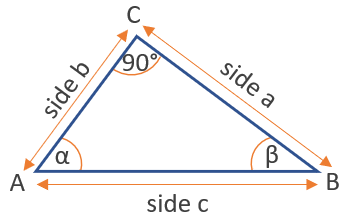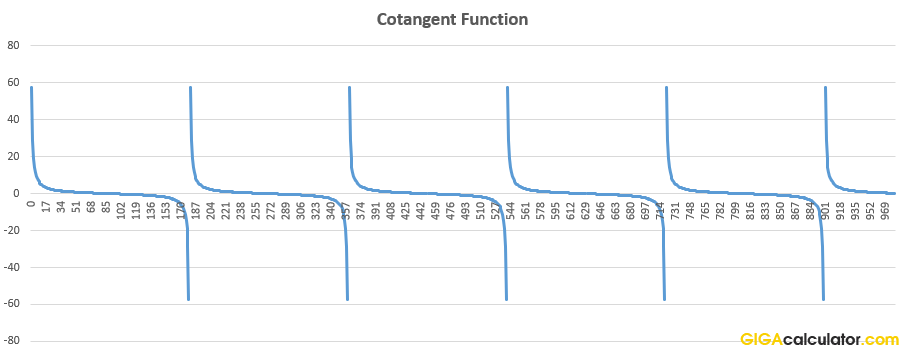# Cotangent Calculator

Use this cotangent calculator to easily calculate the cotangent of an angle given in degrees or radians.

### Calculation results

0 cot(90) =
Share calculator:

Embed this tool:
get code

## Cotangent function ( cot(x) or cotan(x) )

The cotangent is a trigonometric function, defined as the ratio of the length of the side adjacent to the angle to the length of the opposite side, in a right-angled triangle. It is called "cotangent" in reference to its reciprocal - the tangent function - which can be represented as a line segment tangent to a circle.In the figure above, cotα = b / a, and cotβ = a / b. A cotangent of an angle α is also equal to the ratio between its cosine and sine, so cotα = cosα / sinα. Following from the definition, the function results in an undefined value at certain angles, like 0°, 180°, 360°, and so on.

## Related trigonometric functions

The reciprocal of cotangent is the tangent: tan(x), which is the ratio of the length of the opposite side to the length of the side adjacent to the angle.

The inverse of the cotangent is the arccotangent function: arccot(x). It is useful for finding an angle x when cot(x) is known.

## How to calculate the cotangent of an angle?

If you know the angle, then it is a simple calculation of the ratio between the two sides that is required to produce such an angle. Our cotangent calculator accepts input in degrees or radians, so once you have your angle measurement, just type it in and press "calculate".

Alternatively, if the angle is unknown, but the lengths of the two sides of a right angle triangle are known, calculating the cotangent is just a matter of dividing the adjacent by the opposite side. For example, if in the illustration above b = 6 and a = 12, then cot(α) = 6 / 12 = 0.5.

## Applications of the cotangent function

The cotangent function is used in the ASA triangle rule (angle-side-angle). Other than that, it has not had many practical applications since calculators became common, so you should rarely come across it.Above: the cot calculator output for increasing angle values in degrees.

Table of common cotangent values:

Common values of the cotangent function
0 undefined
30° π/6 1.732051
45° π/4 1
60° π/3 0.577350
90° π/2 0
120° 2π/3 -0.577350
135° 3π/4 -1
150° 5π/6 -1.732051
180° π undefined

#### Cite this calculator & page

If you'd like to cite this online calculator resource and information as provided on the page, you can use the following citation:
Georgiev G.Z., "Cotangent Calculator", [online] Available at: https://www.gigacalculator.com/calculators/cot-calculator.php URL [Accessed Date: 01 Apr, 2023].